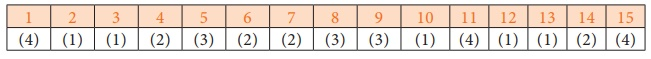Home | | Maths 10th Std | Multiple choice questions

# Multiple choice questions

Mathematics : Mensuration: Multiple choice questions with answers / choose the correct answer with answers - Maths Book back 1 mark questions and answers with solution for Exercise Problems

Multiple choice questions

1. The curved surface area of a right circular cone of height 15 cm and base diameter 16 cm is

(1) 60π cm2

(2) 68π cm2

(3) 120π cm2

(4) 136π cm22. If two solid hemispheres of same base radius r units are joined together along their bases, then curved surface area of this new solid is

(1) 4πr2  sq. units

(2) 6πr2 sq. units

(3) 3πr2  sq. units

(4) 8πr2 sq. units3. The height of a right circular cone whose radius is 5 cm and slant height is 13 cm will be

(1) 12 cm

(2) 10 cm

(3) 13 cm

(4) 5 cm4. If the radius of the base of a right circular cylinder is halved keeping the same height, then the ratio of the volume of the cylinder thus obtained to the volume of original cylinder is

(1) 1:2

(2) 1:4

(3) 1:6

(4) 1:85. The total surface area of a cylinder whose radius is 1/3 of its height isAns: (3)6. In a hollow cylinder, the sum of the external and internal radii is 14 cm and the width is 4 cm. If its height is 20 cm, the volume of the material in it is

(1) 5600π cm3

(2) 11200π cm3

(3) 56π cm3

(4) 3600π cm37. If the radius of the base of a cone is tripled and the height is doubled then the volume is

(4) unchanged8. The total surface area of a hemi-sphere is how much times the square of its radius.

(1) π

(2) 4π

(3) 3π

(4) 2π9. A solid sphere of radius x cm is melted and cast into a shape of a solid cone of same radius. The height of the cone is

(1) 3x cm

(2) x cm

(3) 4x cm

(4) 2x cm10. A frustum of a right circular cone is of height 16cm with radii of its ends as 8cm and 20cm. Then, the volume of the frustum is

(1) 3328π cm3

(2) 3228π cm3

(3) 3240π cm3

(4) 3340π cm311. A shuttle cock used for playing badminton has the shape of the combination of

(1) a cylinder and a sphere

(2) a hemisphere and a cone

(3) a sphere and a cone

(4) frustum of a cone and a hemisphere12. A spherical ball of radius r1 units is melted to make 8 new identical balls each of radius r2 units. Then r1 : r2 is

(1) 2:1

(2) 1:2

(3) 4:1

(4) 1:413. The volume (in cm3) of the greatest sphere that can be cut off from a cylindrical log of wood of base radius 1 cm and height 5 cm is

(1) 4/3 π

(2) 10/3 π

(3) 5 π

(4) 20/3 π14. The height and radius of the cone of which the frustum is a part are h1 units and r1 units respectively. Height of the frustum is h2 units and radius of the smaller base is r2  units. If h2  : h1  = 1 : 2 then r2  : r1  is

(1) 1 : 3

(2) 1 : 2

(3) 2 : 1

(4) 3 : 115. The ratio of the volumes of a cylinder, a cone and a sphere, if each has the same diameter and same height is

(1) 1:2:3

(2) 2:1:3

(3) 1:3:2

(4) 3:1:2